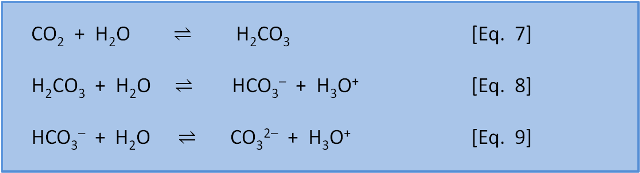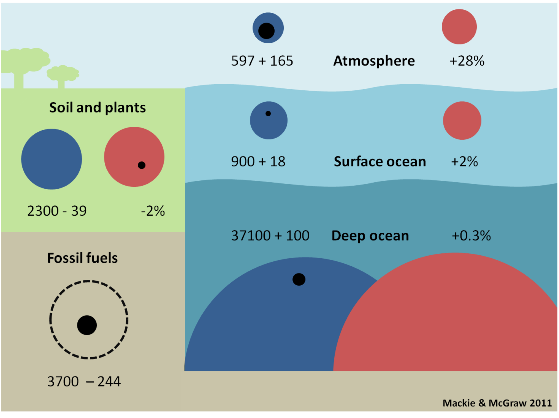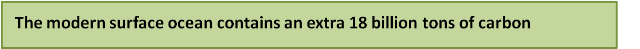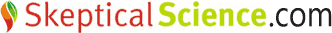# OA not OK part 5: Reservoir dogs

This post is number 5 in a series about ocean acidification. Other posts: Introduction , 1, 2, 3, 4, 5, 6, 7, 8, 9, 10, 11, 12, 13, 14, 15, 16, 17, 18, Summary 1 of 2, Summary 2 of 2.

Welcome to the 5th post in our series about Ocean Acidification. In the last posts, we introduced solubility (post 3) and pH (post 4). We noted that the pH of the surface seawater has dropped from about 8.25 to 8.14 since the pre-industrial revolution, leading to a 29% increase in H3O+. If CO2 emissions continue, a 150% increase in H3O+ is predicted by 2100. Concerns about this increase in acidity are not limited to the decrease in pH (or increase in H3O+), but include the resulting changes to carbon species in seawater.

Just as it is in biology, a species in chemistry is a classification. A chemical species describes different forms a substance can be found in. For example, the species of nitrogen in your garden soil and in stream water may be ammonium (NH4+), ammonia (NH3), and nitrate (NO3-). In the context of ocean acidification we refer to the different species of inorganic carbon – carbon dioxide (CO2), carbonic acid (H2CO3), bicarbonate (HCO3), and carbonate (CO32–) – and how it is possible to interconvert between them.

The equations below describe overall reactions (some are actually made up of several steps) of the inorganic carbon species in seawater. In equation 7, CO2 reacts with water to form carbonic acid (H2CO3). In equation 8, carbonic acid dissociates in water to give bicarbonate (HCO3) and H3O+. In equation 9, bicarbonate dissociates to give carbonate (CO32–) and H3O+.BUT, as we saw in post 1, each equation is in equilibrium and can occur in the left-to-right direction (as written) or the right-to-left direction. That is, the equations do not tell us if a reaction is thermodynamically spontaneous (i.e. which direction is favoured) or about the rate of reaction. These things need to be determined experimentally.

The experiments have, of course, been done. For now we will just note that at typical seawater pH values the reactions in Eq. 7-9. are spontaneous as written from left to right.

Overall equations 7-9 mean that in the oceans 91% of 'carbonate' is in the form of bicarbonate (HCO3), 8% is in the form of carbonate (CO32–), and less than 1% is found as CO2 and H2CO3 (the way we calculate this distribution, the way these reactions have a feedback on each other, and the effect of pH on this balance is discussed in several later posts).

There is a large amount of these inorganic carbon species being held in the ocean. Figure 2 is modified from the IPCC 4th Assessment Report (2007) Figure 7.3 and shows the size of each carbon reservoir on Earth. (In the next series of posts we will discuss the movement of carbon between each reservoir (fluxes) that are in the original figure).Figure 2. Carbon reservoirs as preindustrial size (blue circles) and modern size (red circles) and change, positive or negative (black circles) since the industrial revolution with the change expressed as a % change. Size is measured in gigatons of carbon = Gt C. (1 Gt = 1,000 million tons, i.e. billion tons). Numbers are expressed here as gigatons of carbon (Gt C) because this is the unit used by the IPCC. Other publications may use giga tons of CO2 (Gt CO2); multiply Gt C by 3.67 to convert to Gt CO2 and divide Gt CO2 by 3.67 to get Gt C. Another unit sometimes used is petagrams. 1 Pg = 1 Gt so 1 Pg C = 1 Gt C and 1 Pg CO2 = 1 Gt CO2.

The first number in each box gives the size of the preindustrial reservoir in billions of tons (gigatons, Gt) of carbon (thus, the preindustrial surface ocean contained 900 billion tons of carbon). The second number is the change in the size of the reservoir from preindustrial to modern times in billions of tons of carbon.  NOTE that here 'modern times' means the mid 1990's – the IPCC is very conservative – and the fossil fuel reservoir is an estimate for 'recoverable' fossil fuels.

Thus, the modern surface ocean contains an extra 18 billion tons of carbon, which represents a +2% increase. In contrast, sea life and the surface sediment reservoirs have not changed appreciably in size.Where has so much inorganic carbon in the oceans come from? It has come from the weathering of rocks – the subject of our next post.

Written by Doug Mackie, Christina McGraw , and Keith Hunter . This post is number 5 in a series about ocean acidification. Other posts: Introduction , 1, 2, 3, 4, 5, 6, 7, 8, 9, 10, 11, 12, 13, 14, 15, 16, 17, 18, Summary 1 of 2, Summary 2 of 2.Posted by Doug Mackie on Wednesday, 13 July, 2011The Skeptical Science website by Skeptical Science is licensed under a Creative Commons Attribution 3.0 Unported License.